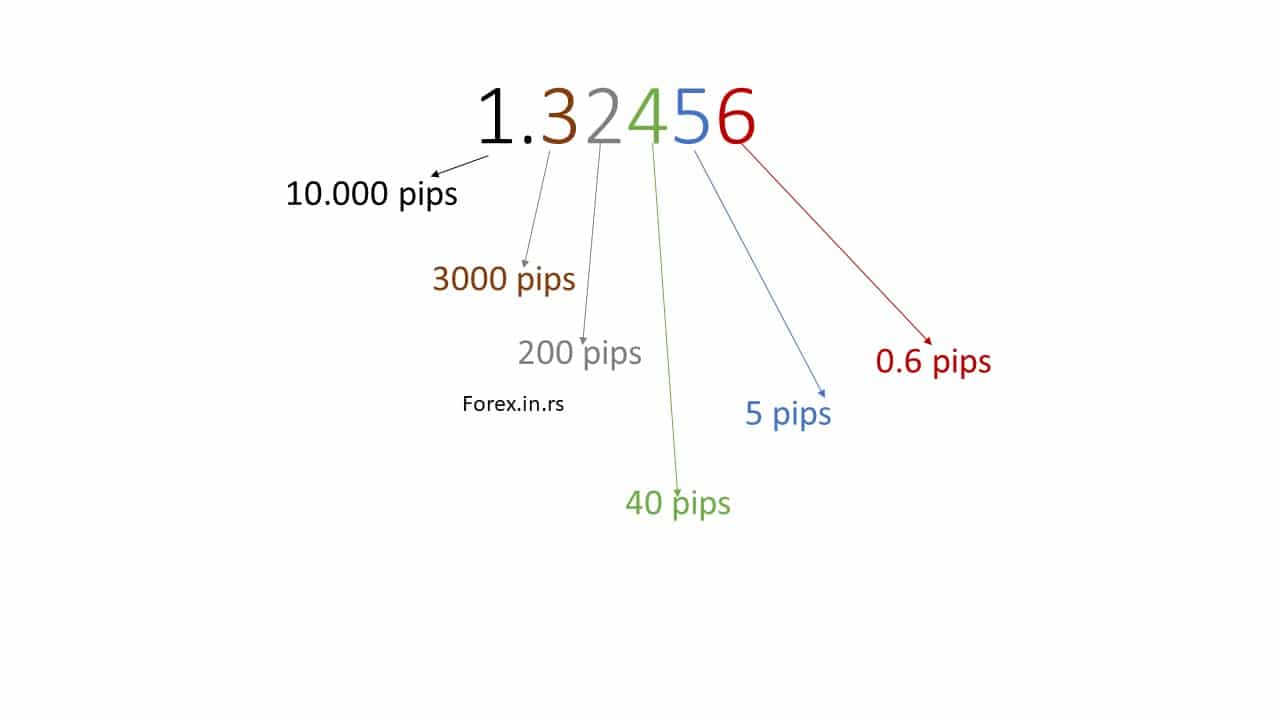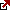# How to Calculate a Pip Value? – Pip Value Formula

Pip and pips are general terms in the trading industry. In this article, we will explain the basic concept.

## What is a pip in forex trading?

PIP is the acronym for the phrase “percentage interest point.” It’s also known as a price interest point. A forex pip is the lowest price increase for a given pair. The pip value is a unit of measurement for currency movement in most currency pairs in the forex trade. The pip between two currencies varies. However, it generally equals the fourth decimal place in most currency pairs. For EURUSD or GBPUSD, for example, 0.0001 is one pip.

Please watch my video to learn how to calculate pip value in dollars for different assets in Metatrader:

The pip value, as you know, is the standard by which a currency pair is compared. This also includes the exchange of currency pairs and the trade size. The significance of pip value is that you can show the amount of exposure and significantly influence your position through pip. The pip value is defined by the pair of currencies, its trade value, and the currency pair’s exchange rate.

To learn how to count pips in the MT4 platform for various symbols, visit our article.

## How to calculate pip difference in forex?

To calculate the pip difference in the forex pair, you need to count the decimal places where the last decimal place represents one pip difference. For example, the EURUSD currency pair exchange rate 1.3012 has four decimal places, and each pip has a value of 0.0001. Therefore, from 1.3012 to 1.3013 is one pip difference.  However, some currency pairs like USDJPY have one pip value of 0.01. For example, from 119.20 to 119.21 is one pip difference.

Now let us calculate the forex pip value:

## Pip Value Formula

To calculate the pip value, multiply the Pip value by the Trade Size and divide the result by the Exchange Rate. This gives you the value of one pip in the quote currency (the second currency in the currency pair).

Pip Value = (Pip x Trade Size) / Exchange Rate

Where:

• Pip is the minor price change a given exchange rate can make, typically the fourth decimal place in most currency pairs (except for the Japanese yen pairs, which use the second decimal place).
• Trade Size refers to the number of units of currency being traded, which is usually expressed in standard lots (100,000 units), mini lots (10,000 units), or micro lots (1,000 units).
• Exchange Rate is the current price of the currency pair being traded, usually quoted to four or five decimal places.

The formula works by calculating the value of one pip in the quote currency (the second currency in the currency pair) and multiplying that by the number of pips gained or lost in the trade. The pip value formula considers the trade size and the currency pair’s exchange rate to do this.

For example, let’s say you’re trading a standard lot of 100,000 units of the EUR/USD currency pair at an exchange rate of 1.1800. If the trade moves in your favor by 50 pips, you would calculate the pip value as follows:

Pip Value = (0.0001 x 100,000) / 1.1800 = 8.47 USD

So for every pip the trade moves in your favor, you would earn or lose USD 8.47. On the other hand, if the trade moves against you by 50 pips, you would lose USD 423.50 (USD 8.47 x 50 pips).

## How to calculate a pip value in forex?

To calculate the pip value, divide one pip (usually 0.0001 for major currencies) by the currency pair’s current value. Then, in the next step, multiply that number by your lot size: the number of base units you are trading.

For example, if the exchange rate is 1.3, the trading size is one lot, and the currency pair EURUSD, then:

Pip Value = (Pip x Trade Size) / Exchange Rate= (0.0001 x 100,000)/1.3= \$13.

The pip (percentage in point) is the smallest unit of measurement in forex trading. It measures the change in the exchange rate of currency pairs. The pip value is the monetary value of one pip, which varies depending on the currency pair being traded, the size of the trade, and the exchange rate.

To calculate the pip value, you need to follow these steps:

1. First, determine the currency pair being traded: EUR/USD.
2. Determine the trade size: 100,000 units of EUR/USD.
3. Finally, determine the exchange rate: For example, 1.1725.
4. Determine the pip value in the quote currency (the second currency in the currency pair): To do this, you need to use the following formula: pip value = (0.0001 / exchange rate) x trade size for the above example, the pip value would be:pip value = (0.0001 / 1.1725) x 100,000 = 8.52 USDThis means that for a 100,000 unit trade of EUR/USD with an exchange rate of 1.1725, each pip is worth USD 8.52.

Note that the pip value will differ for different currency pairs and trades of different sizes and exchange rates.

To simplify it, each Forex account will have a certain number of lots and pips. A lot is a collection or the lowest quantum of a currency you will trade. And the pip is the lowest amount that currency can change.

The value of a foreign currency keeps on varying concerning other currencies. And the absolute value varies with different currencies and with a particular currency. The pip shows to what extent a pair of currencies move up and goes down. The value of a pip, therefore, varies across pairs of currencies. This article discusses pip value and the various aspects that go into calculating the pip value.

If the currency pair’s value increases or goes down 0.0001, the currency pair’s price has gone one pip. Likewise, when the price increases or decreases by 0.0005, the price has moved five pips.## Forex Pip calculator

See more in this Forex Pip Calculator:

Measuring PIP for the different currency

A few currency pairs are expressed in terms of the second digit following the decimal. For example, the pip value of yen-based currency included EUR/JPY, USD/JPY, AUD/JPY, and GBP/JPY using two digits after the decimal.

Thus when the US/JPY goes from 112.00 to 112.90, the price is said to have increased by 90 pips (0.9).

So far, we have defined a pip in forex as the lowest incremental variation in the currency pair price. However, be aware that a pip’s monetary value differs across all pairs of currencies. For this reason, each trader must know the way the pip of the forex currency pair is determined. This helps the money to gain currency and risk management.

## Calculate the pip value in the USD trading account.

The formula to calculate pip value in a USD trading account (or non-JPY account) is : (0.0001 / Current Exchange Rate)

The most traded currency in the international currency market is the US dollar. When the US dollar is listed second in a pair, the pip value is constant and does not have an account financed in USD.

On the other hand, if the USD is listed the other way, that it’s not the second, you need to dive the pip value by the USD/x***rate.

Determining pip from a non-USD trading account

The funded currency account is listed second in a given pair of currencies.

The formula to calculate pip value in non-USD accounts for cross-currency pairs is:

EURJPY Pip Value = [(0.01 / EURJPY Exchange Rate) x (EURUSD Exchange Rate)] x (Units Traded)

YYYJPY Pip Value = [(0.01 / YYYJPY Exchange Rate) x (YYYUSD Exchange Rate)] x (Units Traded)

## Calculate pip value for pairs of other currencies

It is not that many currencies are being traded in your Forex account. Of course, you can have a US account but wish to trade the EUR/GBP (Great Britain Pound).

We will explain how to determine the pip for pairs not included in your currency account.

As you know, the second currency is constant if you have an account in that currency. Thus, the EUR/GBP pip is GBP10 for a standard lot if you have a GBP count.

Make sure to consider the current is proved the pip value: the second currency. Once you know the value, you can determine pip in the particular currency to your desired currency. Then, you can divide it into the PPP/YYY, where PPP is your currency account.

Facts are:

The constant pip amounts are equivalent to \$10 for a standard account.
The constant pip amounts are equal to \$1 for a mini account.
The constant pip amounts are equivalent to \$0.1 for a micro account.

These pip values are coterminous, where USD is listed second. For example, the euro/US dollar, British pound/US dollar, and Australian dollar/USD. New Zealand dollar/USD.

## Pip value Table

Pair Pip value (USD) for 1 lotPip value (EUR) for 1 lotPip value (GBP) for 1 lot
EURUSD 109.168.04
GBPUSD 109.168.04
AUDCHF 11.0710.138.89
AUDHKD 1.271.171.02
AUDJPY 7.66.966.11
AUDNZD 6.265.745.03
AUDSGD 7.526.896.04
AUDUSD 109.168.04
CHFHKD 1.271.171.02
CHFJPY 7.66.966.11
CHFZAR 0.550.50.44
EURAUD 6.686.125.37
EURCHF 11.0710.138.89
EURCZK 0.470.430.37
EURDKK 1.471.341.18
EURGBP 12.4411.3910
EURHKD 1.271.171.02
EURHUF 2.912.662.34
EURJPY 7.66.966.11
EURNOK 0.960.880.77
EURNZD 6.265.745.03
EURPLN 2.332.141.87
EURSEK 0.960.880.77
EURSGD 7.526.896.04
EURTRY 0.520.480.42
EURUSD 109.168.04
EURZAR 0.550.50.44
GBPAUD 6.686.125.37
GBPCHF 11.0710.138.89
GBPHKD 1.271.171.02
GBPJPY 7.66.966.11
GBPNZD 6.265.745.03
GBPPLN 2.332.141.87
GBPSGD 7.526.896.04
GBPUSD 109.168.04
GBPZAR 0.550.50.44
HKDJPY 0.080.070.06
NZDCHF 11.0710.138.89
NZDHKD 1.271.171.02
NZDJPY 7.66.966.11
NZDSGD 7.526.896.04
NZDUSD 109.168.04
SGDCHF 11.0710.138.89
SGDHKD 1.271.171.02
SGDJPY 7.66.966.11
TRYJPY 7.66.966.11
USDCHF 11.0710.138.89
USDCNH 1.461.331.17
USDCZK 0.470.430.37
USDDKK 1.471.341.18
USDHKD 1.271.171.02
USDHUF 2.912.662.34
USDINR 12.0110.999.65
USDJPY 7.66.966.11
USDMXN 0.550.50.44
USDNOK 0.960.880.77
USDPLN 2.332.141.87
USDSAR 2.672.442.14
USDSEK 0.960.880.77
USDSGD 7.526.896.04
USDTHB 29.3426.8723.58
USDTRY 0.520.480.42
USDZAR 0.550.50.44
ZARJPY 7.66.966.11

## Conclusion

If you clearly understand PIP’s notion and how it works, it will help significant benefits; you will be able to judge the quality of the forex market in terms of profitability. Profitability, in turn, is determined by the quality of your judgment and foresight. But, of course, you need to keep abreast of the market: the PIP value of the currency pair and the general market condition around. Thus, calculating a pip value is essential to realize the best result, and you need to leverage it.

You have a PIP calculator in this article, so test it easily using different currency pairs.

Latest posts by Fxigor (see all)

Fxigor

Igor has been a trader since 2007. Currently, Igor works for several prop trading companies. He is an expert in financial niche, long-term trading, and weekly technical levels. The primary field of Igor's research is the application of machine learning in algorithmic trading. Education: Computer Engineering and Ph.D. in machine learning. Igor regularly publishes trading-related videos on the Fxigor Youtube channel. To contact Igor write on: igor@forex.in.rs

Trade gold and silver. Visit the broker's page and start trading high liquidity spot metals - the most traded instruments in the world.Diversify your savings with a gold IRA.

Gold & silver portfolio-building assistance from product professionals.

If you want to trade stocks try: